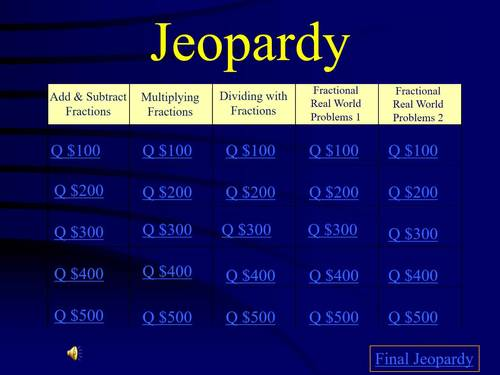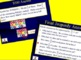# 5th Grade Math Jeopardy- FractionsSubject
Resource Type
File Type

Presentation (Powerpoint) File

(3 MB|54 pages)
Product Rating
Standards
• Product Description
• StandardsNEW

Questions aligned with MAFS (Florida Standards Assessments) item specs, but correlates VERY closely with Common Core Standards. All questions are varying item types- multiple choice, multi-select, open response, table response, equation editor, matching, etc.

Categories are ...

Multiply Fractions

Dividing with Fractions

Fractional Real World Problems 1

Fractional Real World Problems 2

Each category starts off easy and gets harder with higher price values. On each slide, there is an option to click to go to the answer and you can click on Alex Trebec to go back to the game board. View in slideshow, because the answer slides are clickable.

Use for PARCC test prep or FSA test prep OR just as an End of the Year or End of the Unit Review.

Download the preview to explore a question and answer slide from each category then come back to purchase the whole game!

CHECK OUT MY REVIEW PACKETS AS WELL!!!

All 5th Grade Standards ORGANIZED BY STANDARD available HERE

Or if you prefer, I also have the SAME questions with standards mixed together as more of a "Countdown to the Test" packet at 48 Day Countdown to FSA / AIR Test

Other 5th Grade Math Jeopardy Games by Standard

Measurement and Data Jeopardy

Decimal Operations Jeopardy

Place Value and Decimals Jeopardy

Geometry, Order of Operations and Patterns Jeopardy

End of the Year Review

OR GRAB ALL MY 5TH GRADE MATH JEOPARDY GAMES HERE!!!

Like it? Follow my page The Cooperative Teacher

Solve real world problems involving division of unit fractions by non-zero whole numbers and division of whole numbers by unit fractions, e.g., by using visual fraction models and equations to represent the problem. For example, how much chocolate will each person get if 3 people share 1/2 lb of chocolate equally? How many 1/3-cup servings are in 2 cups of raisins?
Interpret division of a whole number by a unit fraction, and compute such quotients. For example, create a story context for 4 ÷ (1/5), and use a visual fraction model to show the quotient. Use the relationship between multiplication and division to explain that 4 ÷ (1/5) = 20 because 20 × (1/5) = 4.
Interpret division of a unit fraction by a non-zero whole number, and compute such quotients. For example, create a story context for (1/3) ÷ 4, and use a visual fraction model to show the quotient. Use the relationship between multiplication and division to explain that (1/3) ÷ 4 = 1/12 because (1/12) × 4 = 1/3.
Apply and extend previous understandings of division to divide unit fractions by whole numbers and whole numbers by unit fractions.
Solve real world problems involving multiplication of fractions and mixed numbers, e.g., by using visual fraction models or equations to represent the problem.
Total Pages
54 pages
Included
Teaching Duration
90 minutes
Report this Resource to TpT
Reported resources will be reviewed by our team. Report this resource to let us know if this resource violates TpT’s content guidelines.